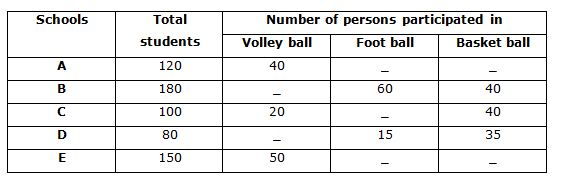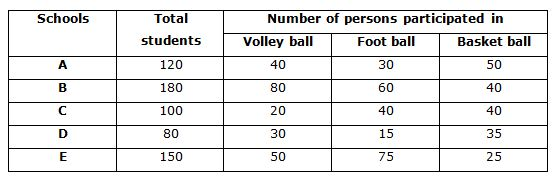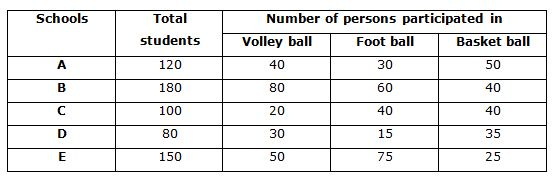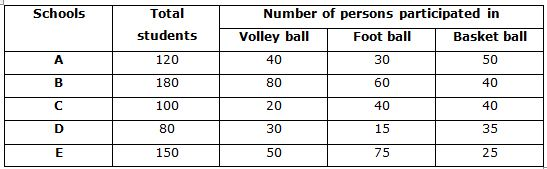# IBPS Clerk Prelims 2018 – Quantitative Aptitude Questions Day-42

Dear Readers, Bank Exam Race for the Year 2018 is already started, To enrich your preparation here we have providing new series of Practice Questions on Quantitative Aptitude – Section. Candidates those who are preparing for IBPS Clerk Prelims 2018 Exams can practice these questions daily and make your preparation effective.

[WpProQuiz 4460]

Directions (1-5): Study the following graph carefully and answer the given questions.

The table shows the number of students participates in different sports in different schools.1) Find the total number of students participated in foot ball from all the given schools, if the ratio of the number of students participated in volley ball to basket ball from schools A and E is 4: 5 and 2: 1 respectively?

a) 200

b) 180

c) 150

d) 220

e) 160

2) What is the difference between the number of students participated in volley ball from schools B and C and number of students participated in basket ball from same schools?

a) 10

b) 20

c) 15

d) 12

e) 25

3) Number of students participated in foot ball from school A and B is approximately what percentage of the number of students participated in basket ball from schools A and E, if the ratio of the number of students participated in volley ball to foot ball from schools A and E is 4: 3 and 2: 3 respectively?

a) 140%

b) 150%

c) 120%

d) 175%

e) 145%

4) What is the ratio of the number of students participated in volley ball from schools C and E to the number of students participated in foot ball from B and D?

a) 14: 15

b) 12: 13

c) 11: 12

d) 9: 8

e) 7: 9

5) Find the ratio of the total students participated from schools A and B to the total students participated from schools C and D.

a) 2: 3

b) 4: 5

c) 3: 7

d) 5: 7

e) None of these

Directions (6 – 10): What value should come in the place of question mark in the given questions?

6) (12*16) + (16*20)+ ? = 1084

a) 448

b) 564

c) 572

d) 640

e) 724

7) 20% of 600 + 45% of 420 = ?

a) 189

b) 224

c) 269

d) 309

e) 289

8) 4/5 of 6/7 of 8/9 of ? = 2880

a) 3460

b) 4725

c) 3280

d) 3180

e) 2890

9) (1073÷29) * (1776/48) * (407/11) = (?)3

a) 37

b) 39

c) 33

d) 42

e) 29

10) 32.56 + 45.24 + 36.89 + 52.67 = ?2 – 1.64

a) 12

b) 11

c) 15

d) 14

e) 13

Directions (1 – 5):Required total = (30+60+40+15+75)

= 220Required difference = (80+20) – (40+40)

= 100 – 80

= 20Required percentage = (30+60)/(50+25) *100

= (90/75) *100

= 120%

Required ratio = (20+50): (60+15)

= 70: 75

= 14: 15

Required ratio = (120+180): (100+80)

= 300: 180

= 5: 3

Directions (6 – 10):

(12*16) + (16*20) + ? = 1084

? = 1084 – 192 – 320

? = 572

20% of 600 + 45% of 420 = ?

1/5 * 600 + 0.45 * 420 = ?

120+189 = ?

309 = ?

4/5 of 6/7 of 8/9 of ? = 2880

? = (2880*5*7*9)/(5*6*8)

? = 4725

(1073÷29) * (1776/48) * (407/11) = (?)3

37 * 37 * 37 = ?3

? = 37

32.56 + 45.24 + 36.89 + 52.67 = ?2 – 1.64

167.36 + 1.64 =?2

169 = ?2

13 =?

Daily Practice Test Schedule | Good Luck

 Topic Daily Publishing Time Daily News Papers & Editorials 8.00 AM Current Affairs Quiz 9.00 AM Current Affairs Quiz (Hindi) 9.30 AM IBPS Clerk Prelims – Reasoning 10.00 AM IBPS Clerk Prelims – Reasoning (Hindi) 10.30 AM IBPS Clerk Prelims – Quantitative Aptitude 11.00 AM IBPS Clerk Prelims – Quantitative Aptitude (Hindi) 11.30 AM Vocabulary (Based on The Hindu) 12.00 PM IBPS Clerk Prelims – English Language 1.00 PM SSC Practice Questions (Reasoning/Quantitative aptitude) 2.00 PM IBPS Clerk – GK Questions 3.00 PM SSC Practice Questions (English/General Knowledge) 4.00 PM Daily Current Affairs Updates 5.00 PM Canara Bank PO Mains – Reasoning 6.00 PM Canara Bank PO Mains – Quantitative Aptitude 7.00 PM Canara Bank PO Mains – English Language 8.00 PM